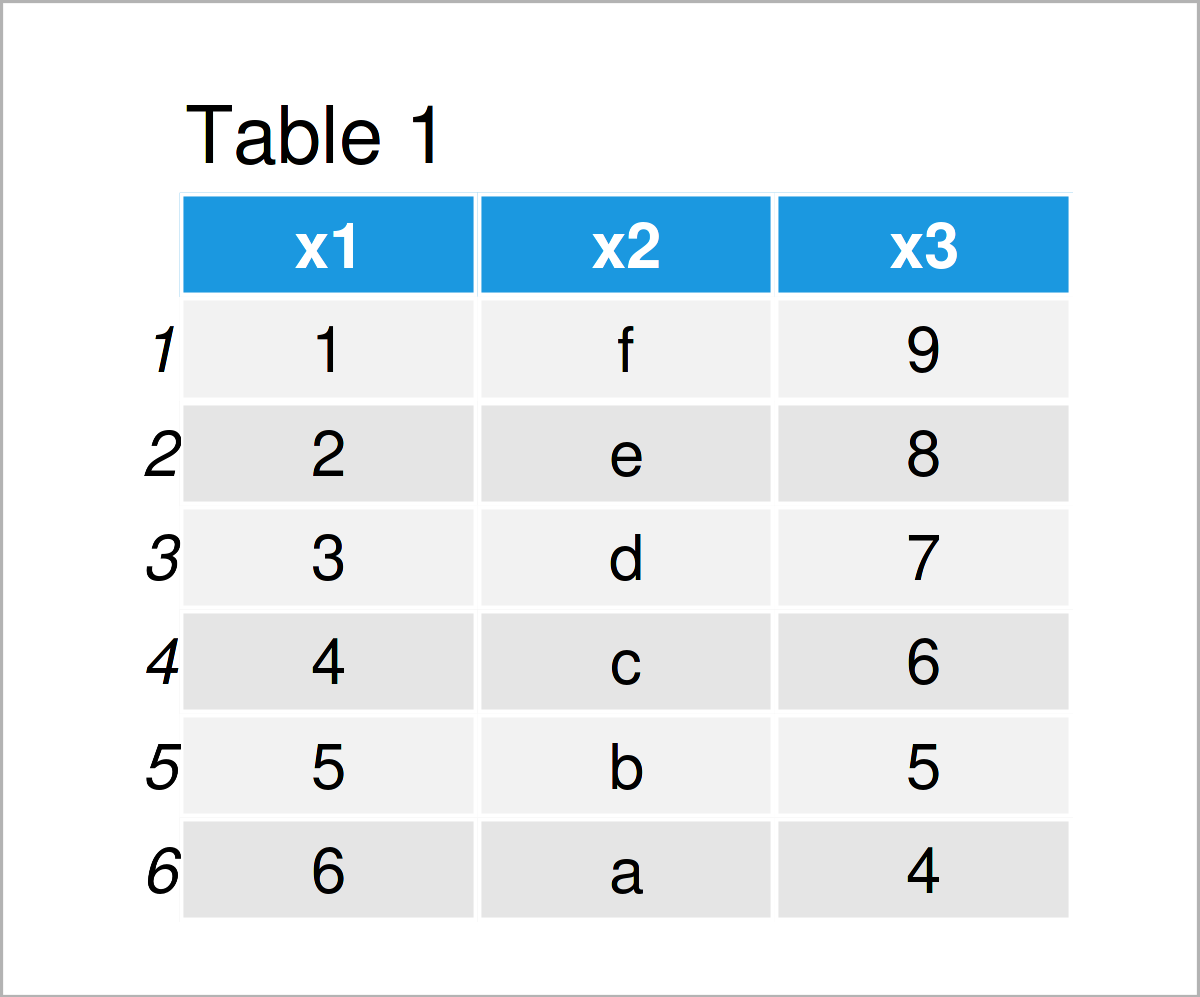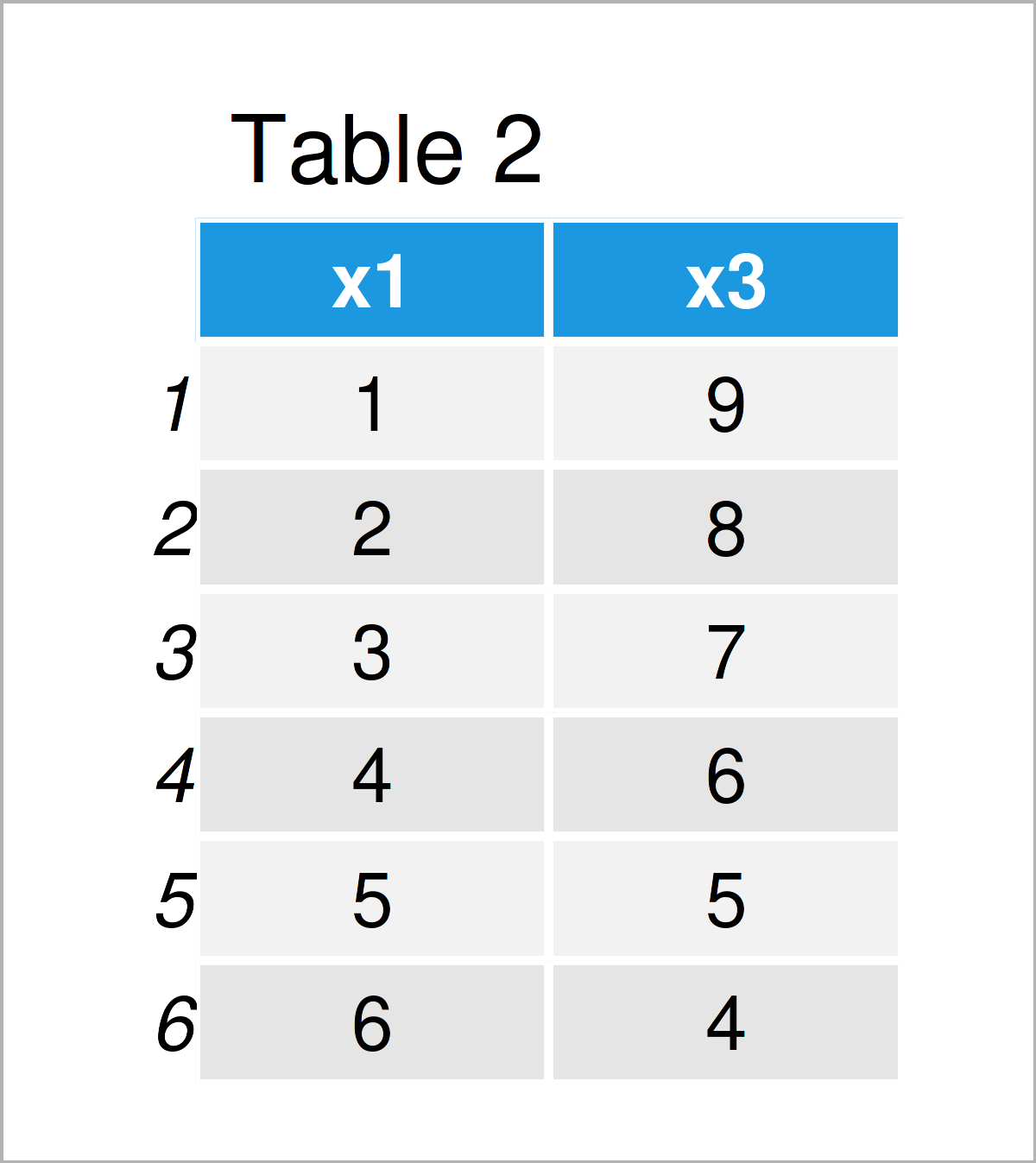# R Error in .subset(x, j) : invalid subscript type ‘list’ (Example)

In this tutorial you’ll learn how to solve the Error in .subset(x, j) : invalid subscript type ‘list’ in the R programming language.

Let’s dive into it:

## Example Data

Consider the following example data:

```data <- data.frame(x1 = 1:6,             # Create example data
x2 = letters[6:1],
x3 = 9:4)
data                                     # Print example data```Table 1 shows that our example data frame contains six observations and the three columns “x1”, “x2”, and “x3”.

## Example 1: Reproduce the Error in .subset(x, j) : invalid subscript type ‘list’

This example demonstrates how to replicate the “Error in .subset(x, j) : invalid subscript type ‘list'”.

Let#s assume that we want to create a subset of our data frame. Then, we might try to select the columns x1 and x3 as shown below:

```data_new <- data[ , list("x1", "x3")]    # Try to subset data
# Error in .subset(x, j) : invalid subscript type 'list'```

As you can see, the RStudio console has returned the “Error in .subset(x, j) : invalid subscript type ‘list'” after executing the previous R code.

The reason for this is that we have tried to extract data frame columns using a list object.

So how can we solve this problem?

## Example 2: Fix the Error in .subset(x, j) : invalid subscript type ‘list’

This section shows how to deal with the “Error in .subset(x, j) : invalid subscript type ‘list'”.

For this, we simply need to exchange the list function by the c function, i.e. we need to subset our data based on a vector instead of a list:

```data_new <- data[ , c("x1", "x3")]       # Properly subset data
data_new                                 # Print updated data```As shown in Table 2, the previous code has created a a new data frame called data_new that contains only a subset of columns from our input data set.

## Video, Further Resources & Summary

I have recently released a video on my YouTube channel, which demonstrates the topics of this article. You can find the video below.

In addition, you could have a look at the other articles on my website:

To summarize: In this tutorial, I have illustrated how to avoid the Error in .subset(x, j) : invalid subscript type ‘list’ in R. In case you have any further questions, don’t hesitate to let me know in the comments below. Furthermore, don’t forget to subscribe to my email newsletter to receive updates on the newest articles.

Subscribe to the Statistics Globe Newsletter